Technical Information Site of Power Supply Design

• Basic Knowledge
• SiC Power Device
• SiC MOSFETs: Behavior of Gate-Source Voltage in a Bridge Configuration
• Behavior of the Gate-Source Voltage During Low-side Switch Turn-off

2021.03.10 SiC Power Device

# Behavior of the Gate-Source Voltage During Low-side Switch Turn-off

SiC MOSFETs: Behavior of Gate-Source Voltage in a Bridge Configuration

In the previous article, the behavior of the gate-source voltage at LS switch turn-on was explained. In this article, the behavior upon LS turn-off is explained in detail.

Behavior of the Gate-Source Voltage During Low-side Switch Turn-off

Below are shown schematically the current behavior in an equivalent circuit when the LS MOSFET is turned off, and waveforms in the circuit. Similarly to during turn-on, the numbers (IV), (V), and (VI) are assigned to correspond to different events. The order of change of VDS and ID is merely different from that during turn-on, and the basic operation is the same. The correspondence with the behavior during turn-on is as follows.

 Turn-off Turn-on Event(IV) → Event(II) Event(V) → Event(III) Event(VI) → Event(I)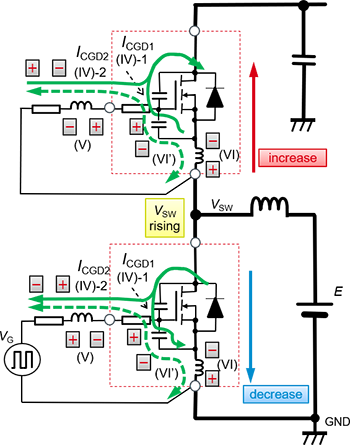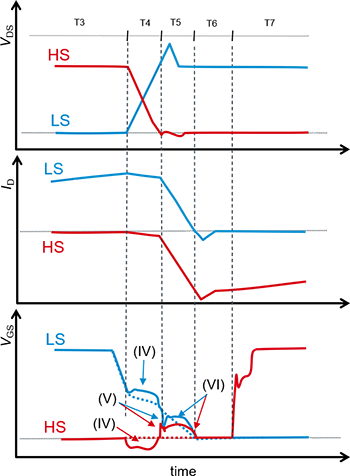The rise in the LS VGS due to dVDS/dt and the minus surge in the HS VGS (T4 in the schematic waveform diagram) are the events (IV).

When, at the end of the period T4 in the schematic waveform diagram, ICGD1 indicated in equation (2) vanishes, the surge that then occurs is event (V). Equation (2) is the same as that presented previously. Thereafter the drain current changes (T6 in the waveform diagram), the emf caused by LSOURCE indicated in equation (1) occurs, and a current flows, indicated as event (VI) in the equivalent circuit. Equation (1) is also the same as that presented previously.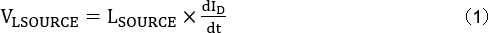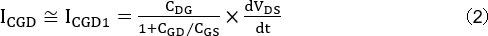This current charges the CGS of the MOSFET with the source side as negative, so that on the HS, VGS is raised, and on the LS, VGS is pulled to the positive side, appearing as an action to impede a fall in VGS. As a result, the VGS behavior seen in the schematic waveform diagram occurs. The dotted line for VGS in the waveform diagram represents the ideal voltage waveform.

Effect of External Gate Resistors

Below we present double-pulse test results when LS is turned off in a SiC MOSFET bridge configuration. The waveform diagram (a) is for the case in which the external gate resistor RG_EXT is 0 Ω, and (b) is for when it is 10 Ω. In the diagrams, (IV), (V), and (VI) are the same as the events explained earlier.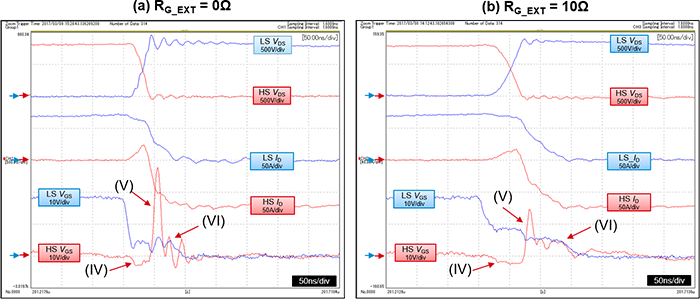As can be seen in the diagrams, the surge in (V) appears prominently.

The influence of the event (IV) due to the change in VDS is minimal, but the minus surge due to event (IV) on the HS frequently exceeds the rated value, and in such cases the circuit must be modified. In order to reduce the HS minus surges upon turn-off, the HS gate resistor RG_EXT is decreased. However, when using a widely employed gate resistor adjustment circuit such as that explained in the previous article, the event (IV) is more pronounced for higher resistance values of RG_ON, so caution must be exercised.

Because the rise in VGS due to event (VI) occurs immediately before the end of turn-off, even when HS has entered turn-on operation, LS is turned off, and essentially no problems result.

#### Key Points:

・When the LS switch has turned off also, the same behavior as during turn-on occurs.

・Minus surges occurring on the HS may exceed the rated value, and in such cases circuit modification is necessary.